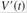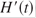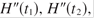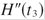### Create an Account

Home / Questions / Water is flowing at a constant rate into a spherical tank. Let V ( t ) be the volume of wa...

# Water is flowing at a constant rate into a spherical tank. Let V ( t ) be the volume of water in the...

Water is flowing at a constant rate into a spherical tank. Let V(t) be the volume of water in the tank and H(t)be the height of the water in the tank at time t.

(a) What are the meanings ofand? Are these derivatives positive, negative, or zero?

(b) Is V”(t) positive, negative, or zero? Explain.

(c) Let t1, t2, and t3 be the times when the tank is one-quarter full, half full, and three-quarters full, respectively. Are the values, , andpositive, negative, or zero? Why?

Apr 24 2020 View more View LessSubscribe To Get Solution